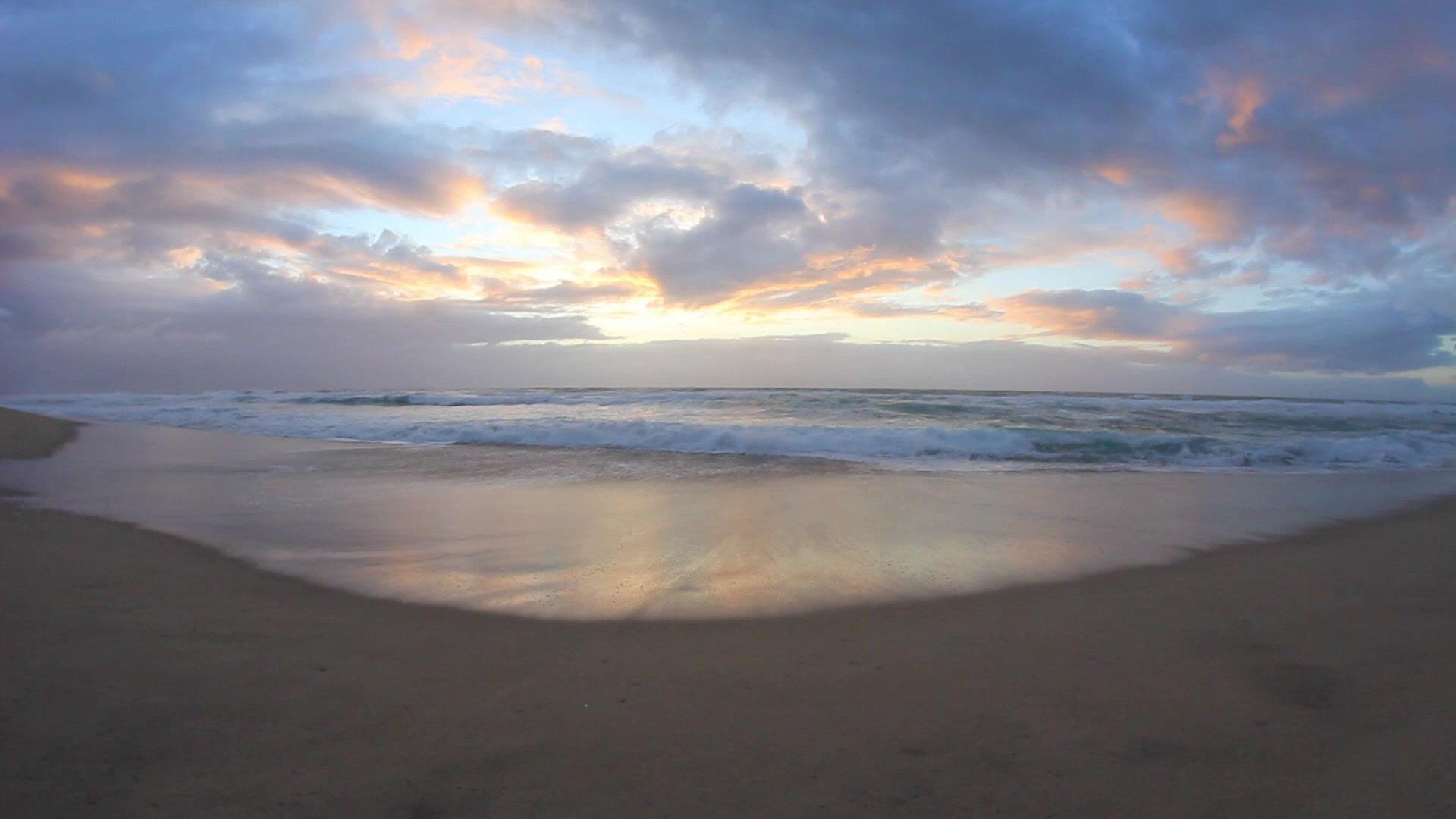top of page# Speed

Speed is a Scalar Quantity.

Speed denotes how fast an object moving.

Its value shows how much distance an object covers for a particular period of time. Here's the formula: Speed = Distance / Time

So if you hear something like "my dad's car was moving at 10km/h" then you instantly know the father's car is a 1971 Ford Pinto. In other words, the higher the value, the faster it is, because it can cover more distance for a particular time, and this also applies vice versa.

And one can rearrange that into this:Cover the quantity you are looking for to get the formula it! Instantaneous Speed

Instantaneous Speed is speed of something at a short period of time.

In other words, it is the speed of something at an instant.

This can be seen in speedometers, where the needle indicates the speed at the exact moment. Usually, the Instantaneous Speed changes during a period of time, but there is also something known as Constant Speed

which basically states a car is moving at the same speed for a period of time. For example, if a car is moving at 80km/h for 5 hours, then it can be stated as moving at a constant speed.

Average Speed

Average speed is a way of showing the speed of a moving body over a period of time. In other words, it tells you how much distance it has cover over a period of time (on average), even if its instantaneous speed varies. We'll get back to that later. The formula for Average Speed is: Total Distance Covered/Total Time Taken

Despite not knowing the instantaneous speed, one can still conclude on a couple of things from the instantaneous speed:

for example, a bus may have multiple speed readings due to slowing down and stopping, but we can still find its average speed and determine is it moving quickly or slowly. We could also figure out approximately how far it moved by multiplying the speed by time, or how long the whole journey took by dividing the distance by the average speed. For example, if we know a bus drove at an average speed of 50km/h, and a full journey across town takes about 5 hours, then we can predict the journey has a length of 250 km.

###### Featured Posts
Check back soon
Once posts are published, you’ll see them here.
No tags yet.